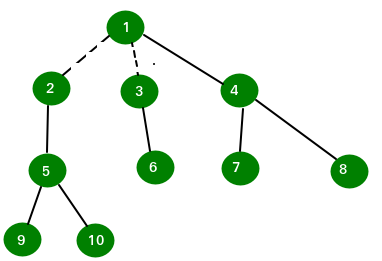# Convert a tree to forest of even nodes

• Difficulty Level : Hard
• Last Updated : 28 Jun, 2021

Given a tree of n even nodes. The task is to find the maximum number of edges to be removed from the given tree to obtain forest of trees having even number of nodes. This problem is always solvable as given graph has even nodes.
Examples:

```Input : n = 10
Edge 1: 1 3
Edge 2: 1 6
Edge 3: 1 2
Edge 4: 3 4
Edge 5: 6 8
Edge 6: 2 7
Edge 7: 2 5
Edge 8: 4 9
Edge 9: 4 10

Output : 2

By removing 2 edges we can obtain the forest with even node tree.``````Dotted line shows removed edges. Any further removal of edge will not satisfy
the even nodes condition.```

Find a subtree with even number of nodes and remove it from rest of tree by removing the edge connecting it. After removal, we are left with tree with even node only because initially we have even number of nodes in the tree and removed subtree has also even node. Repeat the same procedure until we left with the tree that cannot be further decomposed in this manner.
To do this, the idea is to use Depth First Search to traverse the tree. Implement DFS function in such a manner that it will return number of nodes in the subtree whose root is node on which DFS is performed. If the number of nodes is even then remove the edge, else ignore.
Below is implementation of this approach:

## C++

 `// C++ program to find maximum number to be removed``// to convert a tree into forest containing trees of``// even number of nodes``#include``#define N 12``using` `namespace` `std;` `// Return the number of nodes of subtree having``// node as a root.``int` `dfs(vector<``int``> tree[N], ``int` `visit[N],``                      ``int` `*ans, ``int` `node)``{``    ``int` `num = 0, temp = 0;` `    ``// Mark node as visited.``    ``visit[node] = 1;` `    ``// Traverse the adjacency list to find non-``    ``// visited node.``    ``for` `(``int` `i = 0; i < tree[node].size(); i++)``    ``{``        ``if` `(visit[tree[node][i]] == 0)``        ``{``            ``// Finding number of nodes of the subtree``            ``// of a subtree.``            ``temp = dfs(tree, visit, ans, tree[node][i]);` `            ``// If nodes are even, increment number of``            ``// edges to removed.``            ``// Else leave the node as child of subtree.``            ``(temp%2)?(num += temp):((*ans)++);``        ``}``    ``}` `    ``return` `num+1;``}` `// Return the maximum number of edge to remove``// to make forest.``int` `minEdge(vector<``int``> tree[N], ``int` `n)``{``    ``int` `visit[n+2];``    ``int` `ans = 0;``    ``memset``(visit, 0, ``sizeof` `visit);` `    ``dfs(tree, visit, &ans, 1);` `    ``return` `ans;``}` `// Driven Program``int` `main()``{``    ``int` `n = 10;` `    ``vector<``int``> tree[n+2];``    ``tree.push_back(3);``    ``tree.push_back(1);` `    ``tree.push_back(6);``    ``tree.push_back(1);` `    ``tree.push_back(2);``    ``tree.push_back(1);` `    ``tree.push_back(4);``    ``tree.push_back(3);` `    ``tree.push_back(8);``    ``tree.push_back(6);` `    ``tree.push_back(7);``    ``tree.push_back(2);` `    ``tree.push_back(5);``    ``tree.push_back(2);` `    ``tree.push_back(9);``    ``tree.push_back(4);` `    ``tree.push_back(10);``    ``tree.push_back(4);` `    ``cout << minEdge(tree, n) << endl;``    ``return` `0;``}`

## Java

 `// Java program to find maximum number to be removed``// to convert a tree into forest containing trees of``// even number of nodes``import` `java.util.*;` `class` `GFG``{``    ``static` `int` `N = ``12``,ans;``    ` `    ``static` `Vector> tree=``new` `Vector>();``    ` `    ``// Return the number of nodes of subtree having``    ``// node as a root.``    ``static` `int` `dfs( ``int` `visit[], ``int` `node)``    ``{``        ``int` `num = ``0``, temp = ``0``;``    ` `        ``// Mark node as visited.``        ``visit[node] = ``1``;``    ` `        ``// Traverse the adjacency list to find non-``        ``// visited node.``        ``for` `(``int` `i = ``0``; i < tree.get(node).size(); i++)``        ``{``            ``if` `(visit[tree.get(node).get(i)] == ``0``)``            ``{``                ``// Finding number of nodes of the subtree``                ``// of a subtree.``                ``temp = dfs( visit, tree.get(node).get(i));``    ` `                ``// If nodes are even, increment number of``                ``// edges to removed.``                ``// Else leave the node as child of subtree.``                ``if``(temp%``2``!=``0``)``                ``num += temp;``                ``else``                ``ans++;``            ``}``        ``}``    ` `        ``return` `num+``1``;``    ``}``    ` `    ``// Return the maximum number of edge to remove``    ``// to make forest.``    ``static` `int` `minEdge( ``int` `n)``    ``{``        ``int` `visit[] = ``new` `int``[n+``2``];``        ``ans = ``0``;``    ` `        ``dfs( visit, ``1``);``    ` `        ``return` `ans;``    ``}``    ` `    ``// Driven Program``    ``public` `static` `void` `main(String args[])``    ``{``        ``int` `n = ``10``;``        ` `        ``//set the size of vector``        ``for``(``int` `i = ``0``; i < n + ``2``;i++)``        ``tree.add(``new` `Vector());``    ` `        ``tree.get(``1``).add(``3``);``        ``tree.get(``3``).add(``1``);``    ` `        ``tree.get(``1``).add(``6``);``        ``tree.get(``6``).add(``1``);``    ` `        ``tree.get(``1``).add(``2``);``        ``tree.get(``2``).add(``1``);``    ` `        ``tree.get(``3``).add(``4``);``        ``tree.get(``4``).add(``3``);``    ` `        ``tree.get(``6``).add(``8``);``        ``tree.get(``8``).add(``6``);``    ` `        ``tree.get(``2``).add(``7``);``        ``tree.get(``7``).add(``2``);``    ` `        ``tree.get(``2``).add(``5``);``        ``tree.get(``5``).add(``2``);``    ` `        ``tree.get(``4``).add(``9``);``        ``tree.get(``9``).add(``4``);``    ` `        ``tree.get(``4``).add(``10``);``        ``tree.get(``10``).add(``4``);``    ` `        ``System.out.println( minEdge( n));``    ``}``}` `// This code is contributed by Arnab Kundu`

## Python3

 `# Python3 program to find maximum``# number to be removed to convert``# a tree into forest containing trees``# of even number of nodes` `# Return the number of nodes of ``# subtree having node as a root.``def` `dfs(tree, visit, ans, node):``    ``num ``=` `0``    ``temp ``=` `0` `    ``# Mark node as visited.``    ``visit[node] ``=` `1` `    ``# Traverse the adjacency list ``    ``# to find non-visited node.``    ``for` `i ``in` `range``(``len``(tree[node])):``        ``if` `(visit[tree[node][i]] ``=``=` `0``):``            ` `            ``# Finding number of nodes of``            ``# the subtree of a subtree.``            ``temp ``=` `dfs(tree, visit, ans,``                          ``tree[node][i])` `            ``# If nodes are even, increment``            ``# number of edges to removed.``            ``# Else leave the node as child``            ``# of subtree.``            ``if``(temp ``%` `2``):``                ``num ``+``=` `temp``            ``else``:``                ``ans[``0``] ``+``=` `1` `    ``return` `num ``+` `1` `# Return the maximum number of``# edge to remove to make forest.``def` `minEdge(tree, n):``    ``visit ``=` `[``0``] ``*` `(n ``+` `2``)``    ``ans ``=` `[``0``]``    ``dfs(tree, visit, ans, ``1``)` `    ``return` `ans[``0``]` `# Driver Code``N ``=` `12``n ``=` `10` `tree ``=` `[[] ``for` `i ``in` `range``(n ``+` `2``)]``tree[``1``].append(``3``)``tree[``3``].append(``1``)` `tree[``1``].append(``6``)``tree[``6``].append(``1``)` `tree[``1``].append(``2``)``tree[``2``].append(``1``)` `tree[``3``].append(``4``)``tree[``4``].append(``3``)` `tree[``6``].append(``8``)``tree[``8``].append(``6``)` `tree[``2``].append(``7``)``tree[``7``].append(``2``)` `tree[``2``].append(``5``)``tree[``5``].append(``2``)` `tree[``4``].append(``9``)``tree[``9``].append(``4``)` `tree[``4``].append(``10``)``tree[``10``].append(``4``)` `print``(minEdge(tree, n))` `# This code is contributed by pranchalK`

## C#

 `// C# program to find maximum number``// to be removed to convert a tree into``// forest containing trees of even number of nodes``using` `System;``using` `System.Collections.Generic;` `class` `GFG``{``    ``static` `int` `N = 12, ans;``    ` `    ``static` `List> tree = ``new` `List>();``    ` `    ``// Return the number of nodes of``    ``// subtree having node as a root.``    ``static` `int` `dfs(``int` `[]visit, ``int` `node)``    ``{``        ``int` `num = 0, temp = 0;``    ` `        ``// Mark node as visited.``        ``visit[node] = 1;``    ` `        ``// Traverse the adjacency list to``        ``// find non-visited node.``        ``for` `(``int` `i = 0; i < tree[node].Count; i++)``        ``{``            ``if` `(visit[tree[node][i]] == 0)``            ``{``                ``// Finding number of nodes of the``                ``// subtree of a subtree.``                ``temp = dfs(visit, tree[node][i]);``    ` `                ``// If nodes are even, increment number of``                ``// edges to removed.``                ``// Else leave the node as child of subtree.``                ``if``(temp % 2 != 0)``                    ``num += temp;``                ``else``                    ``ans++;``            ``}``        ``}``        ``return` `num + 1;``    ``}``    ` `    ``// Return the maximum number of edge``    ``// to remove to make forest.``    ``static` `int` `minEdge(``int` `n)``    ``{``        ``int` `[]visit = ``new` `int``[n + 2];``        ``ans = 0;``    ` `        ``dfs(visit, 1);``    ` `        ``return` `ans;``    ``}``    ` `    ``// Driver Code``    ``public` `static` `void` `Main(String []args)``    ``{``        ``int` `n = 10;``        ` `        ``//set the size of vector``        ``for``(``int` `i = 0; i < n + 2;i++)``        ``tree.Add(``new` `List<``int``>());``    ` `        ``tree.Add(3);``        ``tree.Add(1);``    ` `        ``tree.Add(6);``        ``tree.Add(1);``    ` `        ``tree.Add(2);``        ``tree.Add(1);``    ` `        ``tree.Add(4);``        ``tree.Add(3);``    ` `        ``tree.Add(8);``        ``tree.Add(6);``    ` `        ``tree.Add(7);``        ``tree.Add(2);``    ` `        ``tree.Add(5);``        ``tree.Add(2);``    ` `        ``tree.Add(9);``        ``tree.Add(4);``    ` `        ``tree.Add(10);``        ``tree.Add(4);``    ` `        ``Console.WriteLine(minEdge(n));``    ``}``}` `// This code is contributed by Rajput-Ji`

## Javascript

 ``

Output:

`2`

Time Complexity: O(n).
Reference:
http://stackoverflow.com/questions/12043252/obtain-forest-out-of-tree-with-even-number-of-nodes
This article is contributed by Anuj Chauhan. If you like GeeksforGeeks and would like to contribute, you can also write an article using write.geeksforgeeks.org or mail your article to review-team@geeksforgeeks.org. See your article appearing on the GeeksforGeeks main page and help other Geeks.